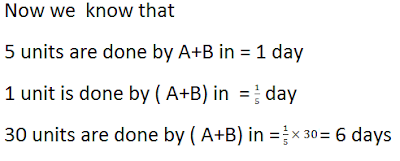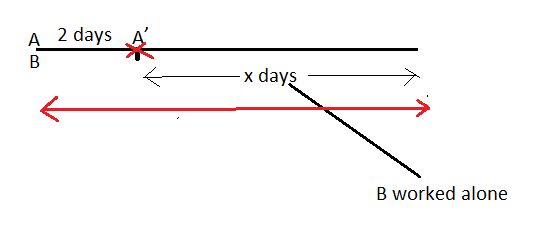New Students Offer - Use Code HELLO

# Time and Work - Shortcut Techniques

Today I am going to share techniques to solve Time and Work question. I have already shared a technique for this chapter here.This topic is based on two main concepts:
• ONE DAY WORK: Unitary Method
• TOTAL WORK
Let us pick up a question to understand it .

Question - A can do a piece of work in 10 days and B can do the same work in 15 days. How long will they take to do the work together.Answer -
A =10 days
B = 15 days
A+B = ? days

To answer we can apply two methods.

### According to the first method

If A can do a piece of work in 10 days

A’s one day work = 1/10

Similarly B’s one day work = 1/15So this method says that

⇒ To do 1/6 work (A+B) take = 1 day

⇒ To do 1 work (A+B) take = 1/ (1/6) = 1 x6/1 = 6 days

Based on this method , the shortcut would be:### SECOND METHOD :

A = 10 days

B  = 15 days

⇒ Total work  = 30 units

WHY 30 units ?

⇒ Remember the LCM of 10 and 15 is 30.

⇒ A does 1 work in 10 days  so in one A will do = 30/10 = 3 units

⇒ B does 1 work in 15 days so in 1 day B will do = 30/15 = 2 units

⇒ So together ( A+B) complete = 3+2 = 5 units in one dayNow which method to be applied, depends upon the kind of

Question - A can do a piece of work in 10 days. A and B together take 6 days to complete the work. How long will B take to complete the work?
Solution -According to the second methodNow according to the third method:⇒ Now the LCM of 10 and 6 is 30 .
Total work = 30 units
⇒ A+B take 6 days to complete 30 units
So in 1 day A+B will complete = 30/6 = 5 units
Similarly A will complete= 30/10 =3 units
⇒ And therefore B will complete  = 5-3 = 2 units
Now B completes 2 units in = 1 day
⇒ B completes 1 unit in = 1/2 day
⇒ B completes 30 units = 1/2×30=15 days

The same concept could be applied in different questions of TIME AND WORK.

Question - A can do a work in 10 days . B can do the same work in 20 days . They both started together but after 2 days A left.The remaining work was done alone by B.How long A and B both took to complete the work?Work done by A in one day = 1/10

⇒So the work done by A in 2 days = 2/10

Work done by B in one day = 1/20

Work done by B in 2 days = 2/20

⇒ Again work done by B in x days = x/20

Together they completed 1 work